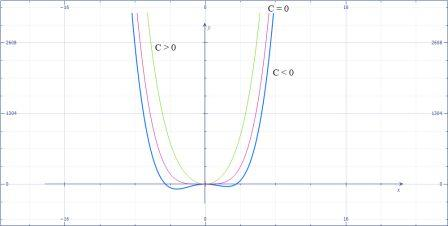# Investigate the family of curves given by f(x) = x^4 + x^3 + cx^2. In particular you should...

## Question:

Investigate the family of curves given by {eq}f(x) = x^4 + x^3 + cx^2. {/eq}

In particular, you should determine the transitional value of at which the number of critical numbers changes and the transitional value at which the number of inflection points changes. Illustrate the various possible shapes with graphs.

## Critical Points and Point of Inflection

To find the desired values of {eq}c {/eq} we need to be familiar with the concepts of the critical number and concept of the points of inflection. We are going to use derivatives to perform the desired calculation.

We have,

{eq}\displaystyle f(x) = x^4 + x^3 + cx^2. {/eq}

• Critical point is a number where the first derivative is either zero or undefined.

Let us find the first derivative,

{eq}\displaystyle f'(x) = 4x^3 + 3x^2 + 2cx {/eq}

Let us find the critical number:

{eq}\displaystyle 4x^3 + 3x^2 + 2cx=0 {/eq}

{eq}\displaystyle x(4x^2 + 3x + 2c)=0 {/eq}

{eq}\displaystyle 9-4(4)(2c)=0 {/eq}

Thus,

{eq}\displaystyle 9=32c {/eq}

{eq}\displaystyle \boxed{\displaystyle c=\frac{9}{32}} {/eq}

Let us find the point of inflection,

{eq}\displaystyle 4x^3 + 3x^2 + 2cx=0 {/eq}

We need to find the second derivative for that,

{eq}\displaystyle f'(x) = 4x^3 + 3x^2 + 2cx {/eq}

{eq}\displaystyle f''(x) = 12x^2 + 6x + 2c {/eq}

• Point of inflection is a point where the second derivative is either 0 or undefined.

{eq}\displaystyle f''(x) = 12x^2 + 6x + 2c=0 {/eq}

{eq}\displaystyle 12x^2 + 6x + 2c=0 {/eq}

In the quadratic equation {eq}ax^2+bx+c=0 {/eq}

Again putting,

{eq}b^2-4ac=0 {/eq}

{eq}\displaystyle 9-4(6)c=0 {/eq}

{eq}\displaystyle \boxed{\displaystyle c=\frac{9}{24}} {/eq}

The graphs below show different value of {eq}c {/eq}.{eq}\displaystyle \text{The blue graph is for the value of C less than 0. The green graph is for the value C greater than 0 and the mid one is for the value of C equals to 0. } {/eq}